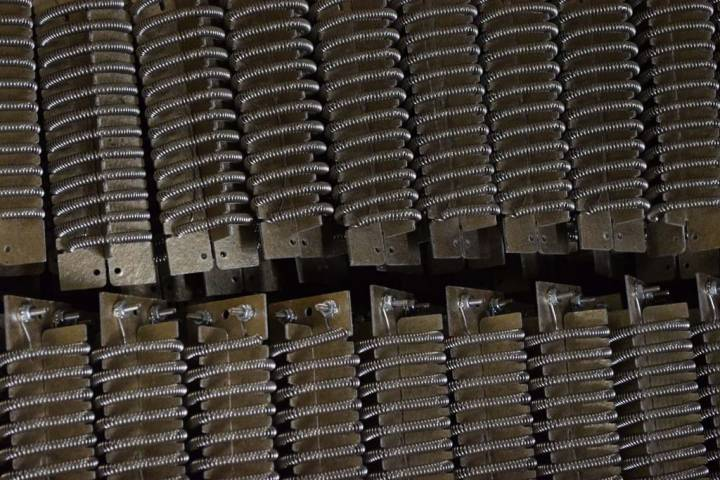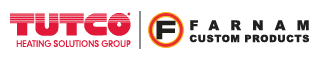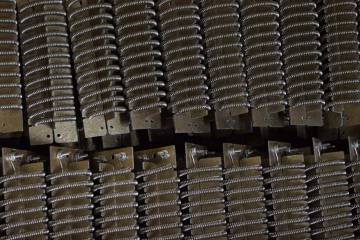Engineer Talk

# How Much Wattage do I Need?By following a few rules-of-thumb you can determine the wattage requirement for your application.

Calculating the wattage requirements to heat a system is a straightforward process as long as all the parameters of heat energy flowing in and out of a system are considered. Heat requirements that must be considered are:

• Initial heat for the startup of the system, usually from ambient temperature to the desired processing temperature.
• Heat losses to the environment due to conduction, convection, and radiation.
• Heating of material being processed during operation.
• Heating of material flowing through the process such as a liquid that will be heated and pumped to be used elsewhere.
• The losses due to phase changes of materials, either during initial heat-up or while processing (melting a solid to a liquid or boiling a liquid to a gas).

Luckily, such precision is usually not required since temperature controllers are commonly employed in most heating applications, meaning that a quick calculation can be used to get you up and running.

Before beginning the calculations, it is important to realize the distinction between energy and power and their relationship to wattage requirements.

• In metric units, power is measured in watts (W) and energy is measured in watt-hours (W x hr).
• In imperial units, power is measured in British Thermal Units per hour (BTU / hr) and energy is measured in BTUs. A BTU is the amount of energy required to heat 1 pound of water by 1°F (specifically from 39° to 40°F).

Think of power as the rate at which energy is used. A 60 watt light bulb uses 60 watt-hours of energy in one hour.

It also should be noted that the difference between the starting temperature and the desired final application temperature is commonly referred to as delta T (ΔT). If a process is started at room temperature, say 72°F, and the application process temperature is 500°F, then ΔT is 500°F - 72°F, or 428°F.

Below are some good guidelines for heating different materials in different situations.

To calculate the wattage requirement to heat steel, use the following equation:

Watts = 0.05 x Lbs of Steel x ΔT (in °F) / Heat-Up Time (in hrs)

Example: To heat 50 lbs of steel by 250°F in 1 hour; .05 x 50 x 250 / 1 = 625 Watts. Using the same example, reaching temperature in 15 minutes (0.25 hrs); .05 x 50 x 250 / .25 = 2,500 Watts. This equation is suitable for mild and stainless steel. If you are heating a different material than steel, you can replace the 0.05 in the equation above with the following coefficients:

• Brass: 0.053
• Aluminum: 0.018
• Copper: 0.056

To calculate the wattage requirement to heat water in a tank, use the following equation:

Watts = 3.1 x Gallons x ΔT (in °F) / Heat-Up Time (in hrs)

Example: To heat 20 gallons of water by 100°F in 30 mins (0.5 hrs); 3.1 x 20 x 100 / 0.5 = 12,400 Watts

To calculate the wattage requirement to heat flowing water, use the following equation:

Watts = 165 x Gallons Per Minute X ΔT (in °F)

Example: To heat water flowing at 2 gallons per minute by 45°F; 165 x 2 x 45 = 14,850 Watts

To calculate the wattage requirement to heat oil in a tank, use the following equation:

Watts = 1.35 x Gallons x ΔT (in °F) / Heat-Up Time (in hrs)

Example: To heat 5 gallons of oil by 300°F in 15 mins (0.25 hrs); 1.35 x 5 x 300 / 0.25 = 8,100 Watts

There are a variety of other equations that can be used to provide good estimates of the power requirement to heat substances in varying situations. The numbers calculated from these formulas do account for typical losses in most applications and will provide a number that is more than adequate to get your process to temperature in the time required.

For more complicated heating applications, the Tutco engineering team can provide support to help with your calculations and provide solutions for your heating needs.

Tutco-Farnam Custom ProductsCalculators
Calculators +